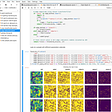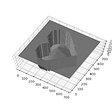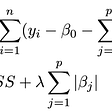# Explaining Bias-Variance Tradeoff to a ML Engineer

Generally data scientists and statisticians are well versed with the term “Bias Variance Tradeoff” as they can very well understand them theoretically as well as from the perspective of many practical algorithms. But to a ML engineer it might be somewhat hard to grasp the mathematical explanations. Here I have tried to capture an intuitive way to explain it with less mathematical notations.

During training and testing of a ML model, it is almost always the case that the loss is never zero.

For e.g. if it’s a binary classification problem with logistic regression, although we train the model on labels 0 and 1, but the model rarely predicts a sigmoid score of 0 or 1 on testing or validation data but most likely 0.23 or 0.89 and so on. Thus even if the model predicts a score of 0.99 for an example with an actual score of 1.0, the loss is still +ve.

The loss/error of the model is decomposed into 3 parts:

Model Error = Noise + Bias + Variance

1. Noise — There are some situations when even with our best possible efforts, we are not able to reduce the loss. This could be due to factors such as incorrect labels, missing features or classes etc. The data scientists could not do anything with their toolset to correct these and only option is probably to get “better” data.
2. Bias — There are some situations where we can reduce the loss simply by tweaking the algorithm or changing the algorithm or running the algorithm for several hours or days. The loss term which can be reduced like this is called the Bias.
3. Variance — There are some situations where although the loss on training data is reducing but loss on validation and/or testing data does not and the loss across different samples of data varies quite a lot. This could be handled by using proper regularization. The loss term which can be reduced like this is called the Variance.

General Rule of Thumb:

`High Bias — Model is UnderfittingHigh Variance — Model is Overfitting`

Some probable reasons for high bias:

• Its a linear algorithm whereas dataset is non linear i.e. if we draw the function of features vs. labels then it is not possible to separate the points corresponding to labels 0 and 1 with a hyperplane.
• Number of features are much less than the number of examples.
• Model not trained to completion or very few epochs were used in training i.e. the loss is far from local or global minima.

Some probable reasons for high variance:

• Linear dataset but non-linear algorithm.
• Very small regularization constant or no regularization at all.
• Number of features much higher than the number of examples.

If you notice points 2 and 3 above you would realize this is a tradeoff.

Lets say that the model has high bias, and in order to lower the bias, the data scientist would probably do one or more of these:

• Change the algorithm to use a more non-linear algorithm i.e. instead of logistic regression probably a deep learning NN.
• Remove any regularization if used.
• Use more features.
• Give more weightages to misclassified examples.
• More epochs during training.

All of the above steps leads to higher probability of a high variance model (overfitting).

The goal of the data scientist is to build a model with low bias and low variance.

Let’s look at how different Tree based ML algorithms handle this tradeoff.

The simplest tree based ML algorithm are Decision Trees.

So if we grow a very deep decision tree i.e. we split a node until there is a single example left, then most likely the tree is overfitting on the training dataset i.e. High Variance.

Conversely if we grow very shallow trees i.e. stop splitting a node at 100 examples, then most likely the tree is underfitted because it could be that out of 100 examples, 50 belong to class 0 and 50 to class 1 and we do not have enough information to make a decision. Here it has High Bias.

One of the strategies to reduce variance is to do averaging, since variance is essentially the error w.r.t the mean.

For e.g. given some random numbers x1, x2, … xn generated from a distribution with mean G, the variance is computed as:

`variance = [(x1-G)^2+(x2-G)^2+ .... + (xn-G)^2]/(n-1)`

Now if we compute ‘m’ different numbers using the following algorithm:

yi = randomly sample k numbers with replacement from xi and take their mean.

With large values of ‘k’, the yi’s are close to the mean of the distribution from which the random numbers were generated i.e. G.

Thus (yi-G) term would be very close to zero and thus the overall variance would also be close to zero.

Also taking an example from Normal distribution.

If the random numbers xi are generated from a Normal distribution N(u, v) with mean u and variance v. Then the average of k normally distributed numbers is a normal distribution with mean u and variance v/k. So variance of yi is v/k.

** If X~N(a1, b1) and Y~N(a2, b2), then pX+qY~N(p*a1+q*a2, p²*b1+q²*b2)

The technique for reducing variance by doing averaging is called Bagging in the ML world.

Random Forest is one such algorithm.

In random forest, we generate an ensemble of decision trees where each decision tree is built using a random sample of the dataset and random sample of features as well to make each tree uncorrelated as much as possible.

The trees are grown to maximum depth so that the bias of each tree to begin with is small but with high variance. Then the variance of the overall system is reduced by doing averaging of predictions.

The predicted score in Random Forest is the average of the individual tree scores.

Important thing to note here is that in order to reduce variance, the samples must be uncorrelated. For e.g. if all predictions from different trees are same then doing an average gives the same score back.

Bagging do not have any effect on Bias.

In order to reduce the Bias, we use another technique known as Boosting.

In boosting, we train a model in multiple rounds:

• In round i, train the model Mi on the dataset Di.
• Use the model Mi to predict on the same dataset Di. Based on the accuracy of the model Mi it is given a weightage of Wi.
• For examples that are misclassified, give them higher weightage or randomly sample more of these examples with probability equal to their weights and create a new dataset Di+1
• Repeat the above steps for Di+1 until the error or loss falls below certain value.
• Final prediction is the weighted average score from all the models i.e. score = W1*score(M1)+W2*score(M2)+…
• Wi+1 ≥ Wi

Note that if we only use the final model during inference, then although it would have the lowest bias but there are high chances it would have high variance.

Thus averaging tackles this problem since averaging reduces variance.

Having weighted averaging tackles the bias part because the model with the lowest bias is given the highest weightage and vice-versa.

Thus boosting strategy helps reduce both Bias and Variance.

Gradient Boosting is one such algorithm.

Since boosting reduces bias and some bit of variance it is advisable to start with a system with low variance and high bias i.e. shallow decision trees and then build a system to reduce the bias.

We can similarly extend this concept to other algorithms too.

--

--

--

## More from Abhijit Mondal

Engineer

Love podcasts or audiobooks? Learn on the go with our new app.

## E-Mail Automation using Python.## Introducing EarthAI Notebook## ML System Design — Language Translation## How to embed Detectron2 in your computer vision project## Elegant Image Resizing with Graph Theory## Named Tuples: A Little Known Machine Learning Helper## Novel techniques to win an Image Classification hackathon (Part-3)## 5 Dangerous Misconceptions about Using Machine Learning in CybersecurityEngineer

## Statistical Learning — Lasso## Probability Questions for ML Interviews## How to Land a Senior Data Scientist Position## Uber Data Scientist Interview Question Walkthrough